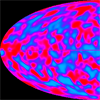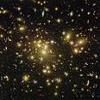#### You may also like### Big and Small Numbers in Physics

Work out the numerical values for these physical quantities.### Big and Small Numbers in the Physical World

Work with numbers big and small to estimate and calculate various quantities in physical contexts.

# Standard Index Form Matching

##### Age 14 to 16 Challenge Level:

$7.5 \times 10^6$ multiplied by $3 \times 10^2$ is the same as $7.5 \times 3 \times 10^6 \times 10^2$

$7.5 \times 10^6$ divided by $3 \times 10^2$ is the same as $\frac{7.5 \times 10^6}{3 \times 10^2}$ which is the same as $\frac{7.5}{3} \times \frac{10^6}{10^2}$